Computer Organization and Structure

Homework #1

Due: 2008/10/7

1.      Given the following three functions:

a.         A two-bit-wide shifter takes two input signals,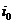and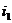, and shifts them to two outputs,and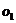, under the control of a shift signal. If this signal SHIFT is false, then the inputs are connected straight through to the outputs. If SHIFT is true, thenis routed toandshould be set to a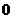.

b.        A two-bit demultiplexer takes an input signal IN and shifts it to one of two outputs,and, under the control of a single SELECT signal. If SELECT is, then IN is connected through toandis connected to a. If SELECT is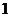, then IN is connected through toandis connected to a.

c.         A two-bit multiplexer takes two input signals,and, and shifts one of them to the single output OUT under the control of a one-bit SELECT signal. If the SELECT signal is false, thenis passed to OUT. If SELECT is true, thenis passed to OUT.

d.        A four-input function that outputs a 1 whenever an odd number of its inputs are 1.

Complete the following four items:

a.         Construct their truth tables.

b.        What are the functions in sum of products forms, using “little m” notation?

c.         Use the Karnaugh map method to simplify the functions in sum of products forms.

d.        Draw logic schematics using AND, OR, and INVERT gates.